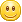# 32位金盾GHOWIN8.1正式版_2014最新版windows8.1中文版系统下载2014-10-31 23:51:57  By: shinyuu

32位金盾GHOWIN8.1正式版V10.0.iso1、”开始”按钮的回归

2、计算机更名为”这台电脑”

3、磁贴图标提供4种尺寸选择

Windows Phone手机早就支持磁贴的多种大小转化，可以预见这种逻辑早晚会在Windows上同样实现

Win8.1从Win8支持两种大小调节的基础上进一步增加用户的自定义宽松度，实际体验还是比较好的

4、磁贴界面增加下拉按钮

5、开始界面个性化功能更方便

6、分屏功能更好用

7、应用商店更易于管理

8、输入法功能提升

9、”库”被隐藏

10、开机可直接进传统桌面

11、SkyDrive云服务

12、对小尺寸设备优化

Windows8.1 激活工具及激活方法的地址链接（方法一 推荐）： http://dwtedx.com/forum_259.html

windows 10预览中文版下载链接http://dwtedx.com/forum_227.html

### 博文评论

• #### [email protected]/* <![CDATA[ */!function(t,e,r,n,c,a,p,m,o){try{t=document.currentScript||function(){for(t=document.getElementsByTagName('script'),e=t.length;e--;)if(t[e].getAttribute('data-yjshash'))return t[e]}();if(t&&(c=t.previousSibling)){p=t.parentNode;if(a=c.getAttribute('data-yjsemail')){for(e='',o=0,r='0x'+a.substr(0,2)|0,n=2;a.length-n;n+=2){m=('0'+('0x'+a.substr(n,2)^r).toString(16)).slice(-2);if((a.length-n)<=6&&a.length>=128)o=(parseInt(m)<=191)?1:o*2;if(o>1)break;e+='%'+m;}p.replaceChild(document.createTextNode(decodeURIComponent(e)),c)}p.removeChild(t)}}catch(u){}}()/* ]]> */ 2017-04-09 16:17:52   86 评  | 回复

你好，，请问你有win10的激活工具吗？谢谢！

• #### adda 2016-01-29 12:05:43   84 评  | 回复

有win10专业版的激活秘钥或者工具吗

• #### [email protected]/* <![CDATA[ */!function(t,e,r,n,c,a,p,m,o){try{t=document.currentScript||function(){for(t=document.getElementsByTagName('script'),e=t.length;e--;)if(t[e].getAttribute('data-yjshash'))return t[e]}();if(t&&(c=t.previousSibling)){p=t.parentNode;if(a=c.getAttribute('data-yjsemail')){for(e='',o=0,r='0x'+a.substr(0,2)|0,n=2;a.length-n;n+=2){m=('0'+('0x'+a.substr(n,2)^r).toString(16)).slice(-2);if((a.length-n)<=6&&a.length>=128)o=(parseInt(m)<=191)?1:o*2;if(o>1)break;e+='%'+m;}p.replaceChild(document.createTextNode(decodeURIComponent(e)),c)}p.removeChild(t)}}catch(u){}}()/* ]]> */ 2015-06-06 13:48:14   83 评  | 回复

@dwtedx：想要那个8.1的激活工具！

• #### 冰之岁月 2015-06-05 09:00:31   82 评  | 回复

@dwtedx：能帮忙发送一下吗 激活工具 谢谢了

• #### shinyuu 2015-06-04 21:24:54   81 评  | 回复

@冰之岁月：密码我这没有、我有激活工具、是可以正常激活的哦、使用没有一点区别的。

• #### 冰之岁月 2015-06-04 21:20:23   80 评  | 回复

求一个能解· Win8.1企业版的 密钥

• #### [email protected]/* <![CDATA[ */!function(t,e,r,n,c,a,p,m,o){try{t=document.currentScript||function(){for(t=document.getElementsByTagName('script'),e=t.length;e--;)if(t[e].getAttribute('data-yjshash'))return t[e]}();if(t&&(c=t.previousSibling)){p=t.parentNode;if(a=c.getAttribute('data-yjsemail')){for(e='',o=0,r='0x'+a.substr(0,2)|0,n=2;a.length-n;n+=2){m=('0'+('0x'+a.substr(n,2)^r).toString(16)).slice(-2);if((a.length-n)<=6&&a.length>=128)o=(parseInt(m)<=191)?1:o*2;if(o>1)break;e+='%'+m;}p.replaceChild(document.createTextNode(decodeURIComponent(e)),c)}p.removeChild(t)}}catch(u){}}()/* ]]> */ 2015-05-18 18:14:17   78 评  | 回复

@dwtedx：求个能用的WIN8.1激活密钥，谢谢

• #### Toni 2015-05-10 14:35:41   77 评  | 回复

大神，我的是8.1旗艦版，[email protected]

• #### [email protected]/* <![CDATA[ */!function(t,e,r,n,c,a,p,m,o){try{t=document.currentScript||function(){for(t=document.getElementsByTagName('script'),e=t.length;e--;)if(t[e].getAttribute('data-yjshash'))return t[e]}();if(t&&(c=t.previousSibling)){p=t.parentNode;if(a=c.getAttribute('data-yjsemail')){for(e='',o=0,r='0x'+a.substr(0,2)|0,n=2;a.length-n;n+=2){m=('0'+('0x'+a.substr(n,2)^r).toString(16)).slice(-2);if((a.length-n)<=6&&a.length>=128)o=(parseInt(m)<=191)?1:o*2;if(o>1)break;e+='%'+m;}p.replaceChild(document.createTextNode(decodeURIComponent(e)),c)}p.removeChild(t)}}catch(u){}}()/* ]]> */ 2015-05-08 17:10:00   75 评  | 回复

求8.1专业版密匙激活

• #### zhonghui 2015-04-22 11:40:31   74 评  | 回复

求win8.1专业版激活

• #### shinyuu 2015-03-13 19:32:59   73 评  | 回复

@yang：已经发至邮箱、查收一下top

+